Demonstration of the orthogonality of the trigonometric functions

Integrating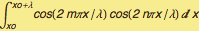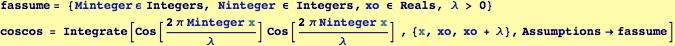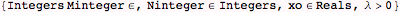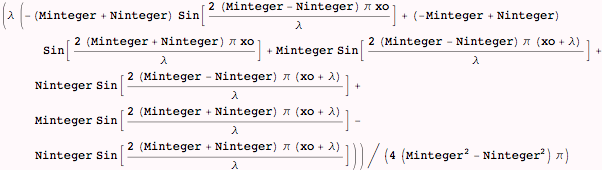Demonstrating that the integral goes to zero for m≠n and λ/2 if m=n (we demonstrate this by using random choices for m and n)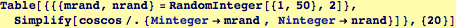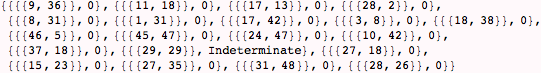If we run the above command enough times, we will see  that results where n=m give indeterminate values, for these we should use a Limit.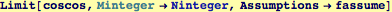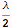Performing the same steps for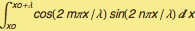and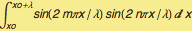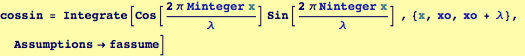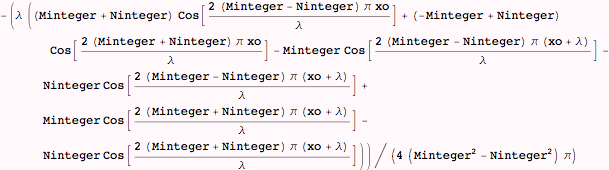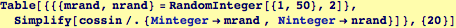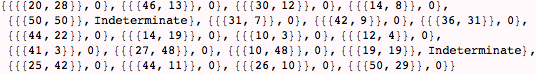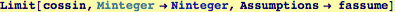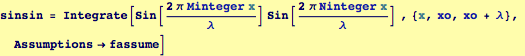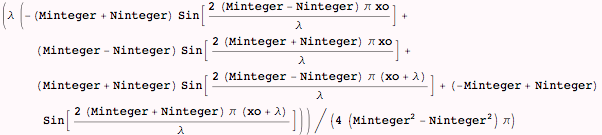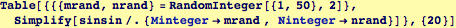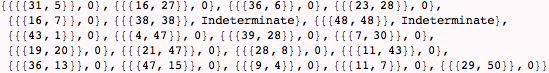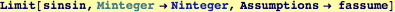Created by Wolfram Mathematica 6.0  (24 October 2007)